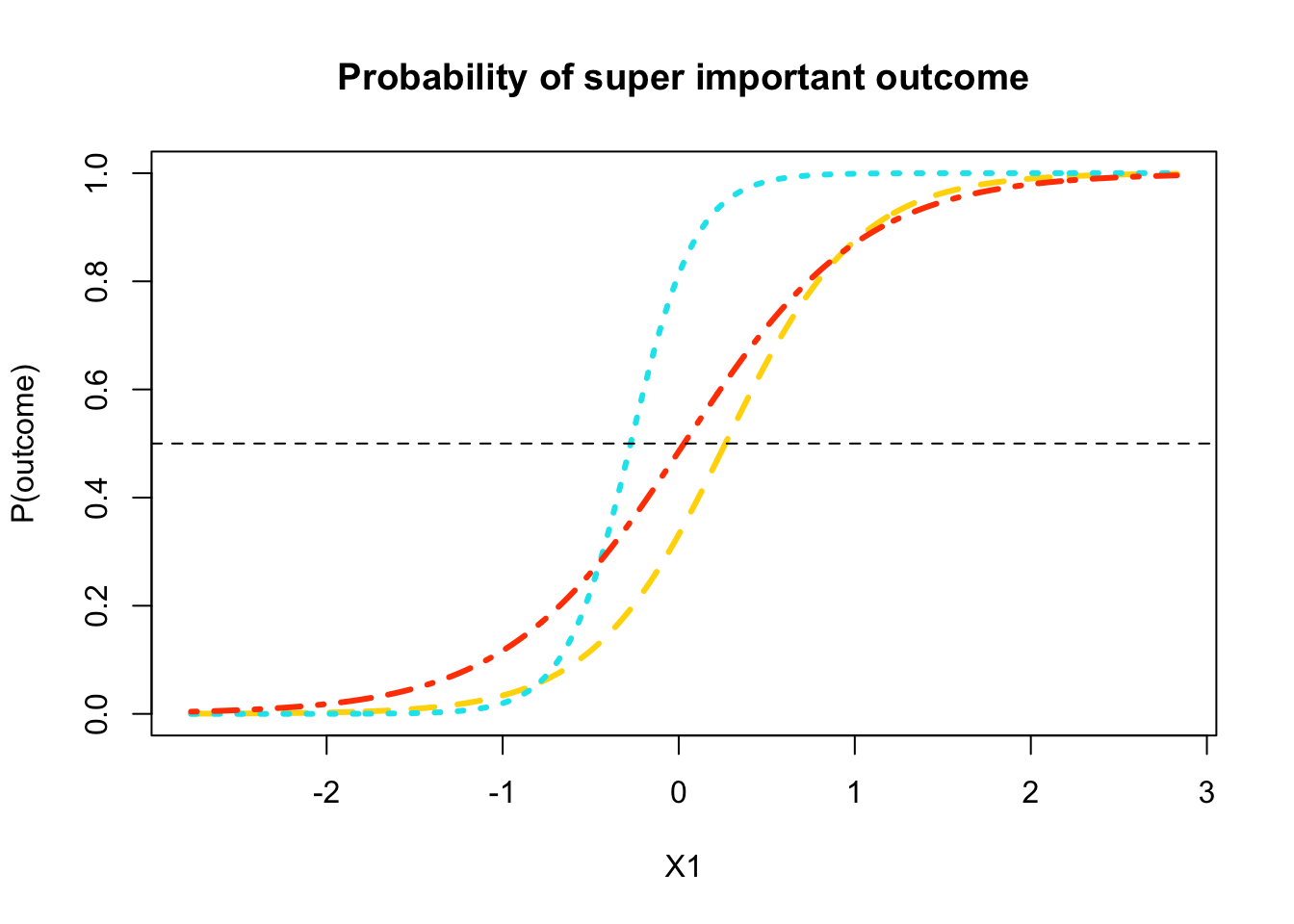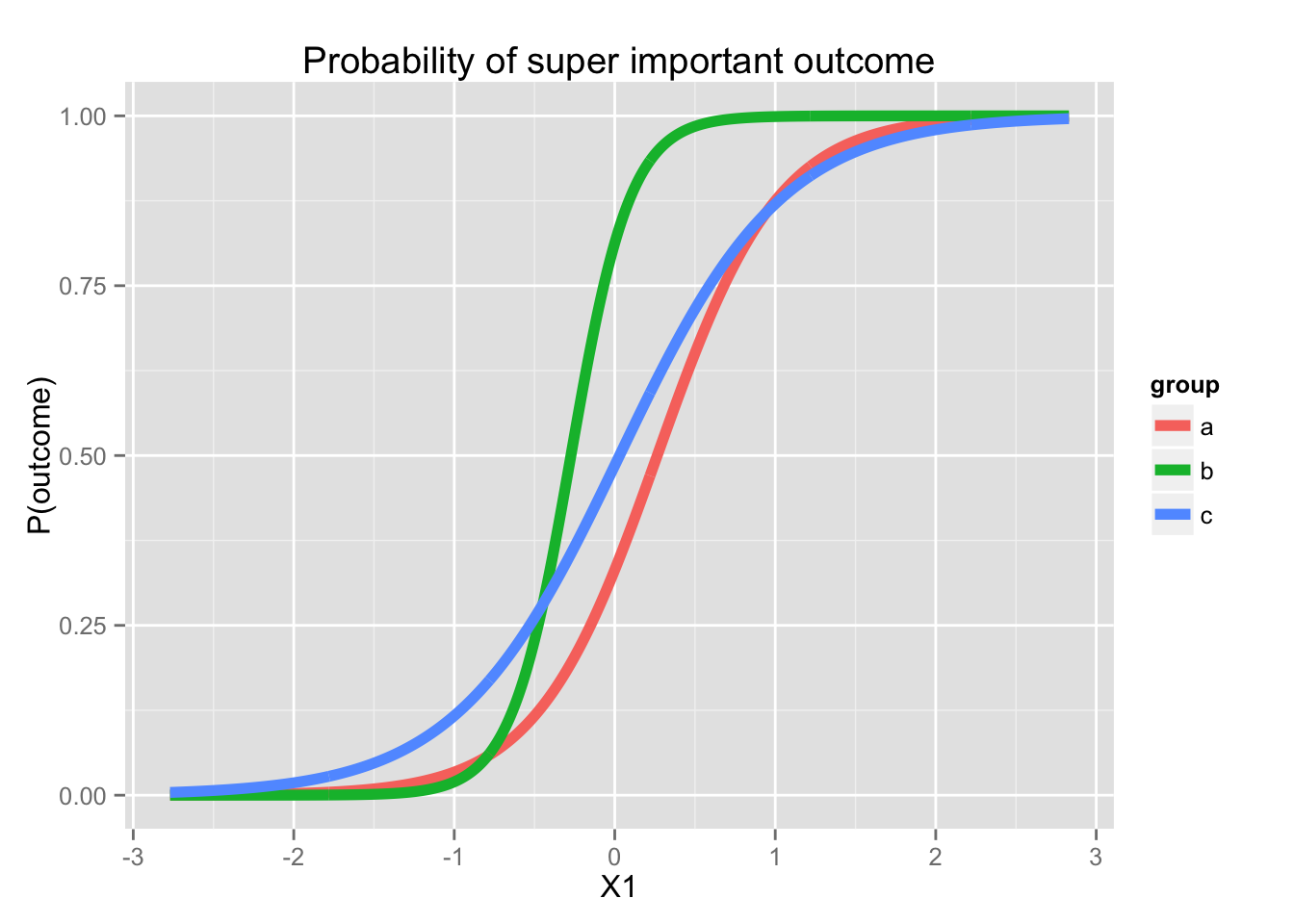# Plotting your logistic regression models

This code is all available on Rose’s github: https://github.com/rosemm/rexamples/blob/master/logistic_regression_plotting_part1.Rmd

# Plotting the results of your logistic regression Part 1: Continuous by categorical interaction

We’ll run a nice, complicated logistic regresison and then make a plot that highlights a continuous by categorical interaction.

set.seed(24601) # setting this so the random results will be repeatable

library(MASS)
covmat <- matrix(c(1.0,   0.2,   0.6,
0.2,   1.0,  -0.5,
0.6,  -0.5,   1.0), nrow=3) # the true cov matrix for my data
data <- mvrnorm(300, mu=c(0,0,0), Sigma=covmat) # generate random data that match that cov matrix
colnames(data) <- c("X1", "X2", "DV")
data <- as.data.frame(data)
data$group <- gl(n=3, k=ceiling(nrow(data)/3), labels=c("a", "b", "c", "d")) # add some group differences and interaction stuff... data$DV <- with(data, ifelse(group=="c" & X1 > 0, DV+rnorm(n=1, mean=1),
ifelse(group=="b" & X1 > 0, DV+rnorm(n=1, mean=2) , DV)))
# make DV binary
data$DV <- ifelse(data$DV > 0, 1, 0)
head(data)
##            X1         X2 DV group
## 1  0.75191377  0.2978933  1     a
## 2 -1.45574399 -0.6868934  0     a
## 3 -0.07297941  0.2450438  0     a
## 4  0.91090328 -1.6934376  1     a
## 5  1.19905258  0.4765307  0     a
## 6  0.42466903 -0.9937526  1     a

## Get the coefficients from your logistic regression model

First, whenever you’re using a categorical predictor in a model in R (or anywhere else, for that matter), make sure you know how it’s being coded!! For this example, we want it dummy coded (so we can easily plug in 0’s and 1’s to get equations for the different groups). This is called contr.treatment() in R.

contrasts(data$group) ## b c d ## a 0 0 0 ## b 1 0 0 ## c 0 1 0 ## d 0 0 1 # Great, that's what we wanted. And we can see that a is the reference group. # If you want to change what contrasts will run, you can add an argument to the glm() call. For example contrasts="contr.treatment" will make it traditional dummy coding if it isn't already. Now we can run that model. # note this use of exponent in a formula will give us all 2-way interactions model <- glm(DV ~ (X1 + X2 + group)^2, data=data, na.action="na.exclude", family="binomial") summary(model) ## ## Call: ## glm(formula = DV ~ (X1 + X2 + group)^2, family = "binomial", ## data = data, na.action = "na.exclude") ## ## Deviance Residuals: ## Min 1Q Median 3Q Max ## -2.44094 -0.45991 0.04136 0.52301 2.74705 ## ## Coefficients: ## Estimate Std. Error z value Pr(>|z|) ## (Intercept) -0.5873 0.3438 -1.708 0.087631 . ## X1 2.6508 0.5592 4.740 2.13e-06 *** ## X2 -2.2599 0.4977 -4.540 5.61e-06 *** ## groupb 2.2111 0.5949 3.717 0.000202 *** ## groupc 0.6650 0.4131 1.610 0.107456 ## X1:X2 0.1201 0.2660 0.452 0.651534 ## X1:groupb 2.7323 1.2977 2.105 0.035253 * ## X1:groupc -0.6816 0.7078 -0.963 0.335531 ## X2:groupb 0.8477 0.7320 1.158 0.246882 ## X2:groupc 0.4683 0.6558 0.714 0.475165 ## --- ## Signif. codes: 0 '***' 0.001 '**' 0.01 '*' 0.05 '.' 0.1 ' ' 1 ## ## (Dispersion parameter for binomial family taken to be 1) ## ## Null deviance: 412.88 on 299 degrees of freedom ## Residual deviance: 205.46 on 290 degrees of freedom ## AIC: 225.46 ## ## Number of Fisher Scoring iterations: 7 model$coef
## (Intercept)          X1          X2      groupb      groupc       X1:X2
##  -0.5872841   2.6508212  -2.2599250   2.2110951   0.6649971   0.1201166
##   X1:groupb   X1:groupc   X2:groupb   X2:groupc
##   2.7323113  -0.6816327   0.8476695   0.4682893
# save the coefficient values so we can use them in the equations
b0 <- model$coef # intercept X1 <- model$coef
X2 <- -model$coef groupb <- model$coef
groupc <- model$coef X1.X2 <- model$coef
X1.groupb <- model$coef X1.groupc <- model$coef
X2.groupb <- model$coef X2.groupc <- model$coef

Note: If you were working in SPSS (or for some other reason you have run a model but can’t generate a plot for it), you can enter in your coefficients here, like this:

b0 <- -0.5872841 # intercept
X1 <- 2.6508212
X2 <- -2.2599250
groupb <- 2.2110951
groupc <- 0.6649971
X1.X2 <- 0.1201166
X1.groupb <- 2.7323113
X1.groupc <- -0.6816327
X2.groupb <- 0.8476695
X2.groupc <- 0.4682893

## Calculate probabilities for the plot

First, decide what variable you want on your x-axis. That’s the only variable we’ll enter as a whole range. (The range we set here will determine the range on the x-axis of the final plot, by the way.)

X1_range <- seq(from=min(data$X1), to=max(data$X1), by=.01)

Next, compute the equations for each group in logit terms. These equations need to include every coefficient for the model you ran. You’ll need to plug in values for all but one variable – whichever variable you decided will be displayed on the x-axis of your plot. You make a separate equation for each group by plugging in different values for the group dummy codes.

X2_val <- mean(data\$X2) # by plugging in the mean as the value for X2, I'll be generating plots that show the relationship between X1 and the outcome "for someone with an average X2".

a_logits <- b0 +
X1*X1_range +
X2*X2_val +
groupb*0 +
groupc*0 +
X1.X2*X1_range*X2_val +
X1.groupb*X1_range*0 +
X1.groupc*X1_range*0 +
X2.groupb*X2_val*0 +
X2.groupc*X2_val*0 # the reference group

b_logits <- b0 +
X1*X1_range +
X2*X2_val +
groupb*1 +
groupc*0 +
X1.X2*X1_range*X2_val +
X1.groupb*X1_range*1 +
X1.groupc*X1_range*0 +
X2.groupb*X2_val*1 +
X2.groupc*X2_val*0

c_logits <- b0 +
X1*X1_range +
X2*X2_val +
groupb*0 +
groupc*1 +
X1.X2*X1_range*X2_val +
X1.groupb*X1_range*0 +
X1.groupc*X1_range*1 +
X2.groupb*X2_val*0 +
X2.groupc*X2_val*1

# Compute the probibilities (this is what will actually get plotted):
a_probs <- exp(a_logits)/(1 + exp(a_logits))
b_probs <- exp(b_logits)/(1 + exp(b_logits))
c_probs <- exp(c_logits)/(1 + exp(c_logits))

## Plot time!

# We'll start by plotting the ref group:
plot(X1_range, a_probs,
ylim=c(0,1),
type="l",
lwd=3,
lty=2,
col="gold",
xlab="X1", ylab="P(outcome)", main="Probability of super important outcome")

# Add the line for people who are in the b group
lines(X1_range, b_probs,
type="l",
lwd=3,
lty=3,
col="turquoise2")

# Add the line for people who are in the c group
lines(X1_range, c_probs,
type="l",
lwd=3,
lty=4,
col="orangered")

# add a horizontal line at p=.5
abline(h=.5, lty=2)Or, you can do it in ggplot2!

library(ggplot2); library(tidyr)
# first you have to get the information into a long dataframe, which is what ggplot likes :)
plot.data <- data.frame(a=a_probs, b=b_probs, c=c_probs, X1=X1_range)
plot.data <- gather(plot.data, key=group, value=prob, a:c)
head(plot.data)
##          X1 group         prob
## 1 -2.770265     a 0.0003264137
## 2 -2.760265     a 0.0003351590
## 3 -2.750265     a 0.0003441385
## 4 -2.740265     a 0.0003533586
## 5 -2.730265     a 0.0003628255
## 6 -2.720265     a 0.0003725460
ggplot(plot.data, aes(x=X1, y=prob, color=group)) + # asking it to set the color by the variable "group" is what makes it draw three different lines
geom_line(lwd=2) +
labs(x="X1", y="P(outcome)", title="Probability of super important outcome")1. Aaron Charlton

Best. Post. Ever. Never take this down — please!

2. R B

awesome. nice post

3. Martin

Excellent post. I have been looking for such a clear explanation for hours. I have only one quick follow-up question: Could you (or anybody else) explain me how one can add a legend to the first plot?

• rosem@uoregon.edu

Thanks! To add a legend to a base R plot (the first plot is in base R), use the function legend. You have to enter all of the information for it (the names of the factor levels, the colors, etc.) manually. Here’s a nice tutorial . If you use the ggplot2 code instead, it builds the legend for you automatically.

• Martin

Thank you very much for the quick answer.

4. Carter

Nice plots. It would be nice if you would add a nice real world interpretation of each line. This plot is useful but explain it in plain language to someone with less maths background and you have a winner in the business world. How do the groups compare to each other? What does a faster vertical slope mean? etc.

5. Laura Picchi

Hi, this is a really useful post! Anyway I would like to know if this script can be used even with mixed effects models (glmer formula).